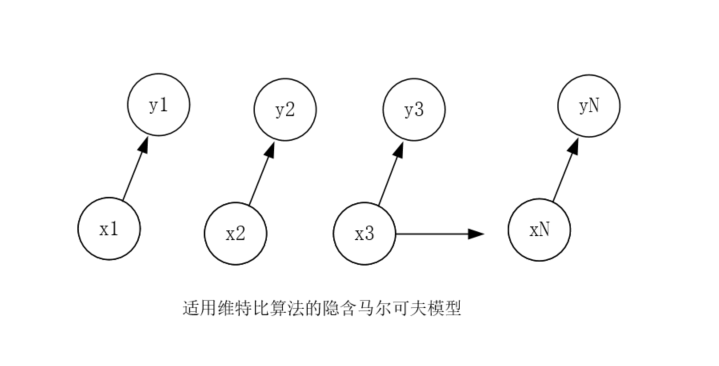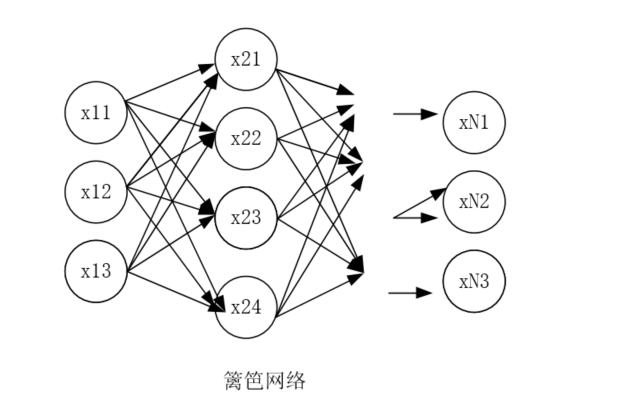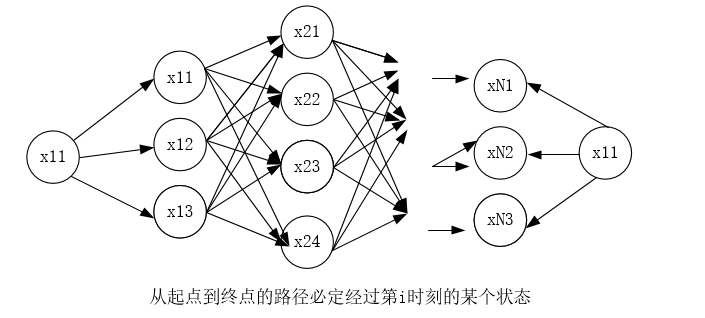• viterbi算法

2015-05-08 20:45:53
viterbi decode matlab代码； 包括自己实现的matlat viterbi 译码和调用matlab内部模块的代码
• 代码中有示例，来自李航《统计学习方法》function [Delta,Psi,P,I] = Viterbi(A,B,Pi,O)% 函数功能：利用维特比算法找到观测序列O的最优路径%% 参考文献：李航《统计学习方法》%% 思路：% 1，初始化% delta_1(i) = ...

维特比算法实际是用动态规划求解隐马尔可夫模型解码问题，即用动态规划求概率最大路径(最优路径)。代码中有示例，来自李航《统计学习方法》

function [Delta,Psi,P,I] = Viterbi(A,B,Pi,O)

% 函数功能：利用维特比算法找到观测序列O的最优路径

%

% 参考文献：李航《统计学习方法》

%

% 思路：

% 1，初始化

% delta_1(i) = Pi_i * b_i(o1), i = 1,2,...,N

% psi_1(i) = o, i = 1,2,...,N

% 2,递推，对于t = 2,3,...,T

% delta_t(i) = max_1-from-1-to-N(delta_t-1(j) * a_ji) * b_i(ot), i = 1,2,...,N

% psi_t(i) = arg max_1-from-1-to-N(delta_t-1(j) * a_ji), i = 1,2,...,N

% 3,终止

% 最优路径概率P* = max_1-from-1-to-N(delta_T(i))

% 最优路径终点i*_T = arg max_1-from-1-to-N(delta_T(i))

% 4,最优路径回溯，对于t = T-1,T-2,...,1

% i*_t = psi_t+1(i*_t+1)

% 最优路径I* = (i*_1,i*_2,...,i*_T)

% 【提示：“思路”中的i,j和本程序中的i,j不存在对应关系，只是方便理解原理】

%

% 输入：模型参数A,B,Pi，观测序列O

% 输出：Delta矩阵：在时刻t状态为i的所有单个路径中概率最大值构成的矩阵，

% 为N*K阶，即行数表示状态数，列数表示时刻。

% Psi矩阵：在时刻t状态为i的所有单个路径中概率最大路径的第t-1个结点构成的矩阵，

% 为N*K阶，即行数表示状态数，列数表示时刻。且第一列为0。

% P：观测序列O的最优路径概率。

% I：观测序列O的最优路径。

%

% 示例：(李航《统计学习方法》P186例10.3)

% 输入

% A =

% 0.5000 0.2000 0.3000

% 0.3000 0.5000 0.2000

% 0.2000 0.3000 0.5000

% B =

% 0.5000 0.5000

% 0.4000 0.6000

% 0.7000 0.3000

% Pi =

% 0.2000

% 0.4000

% 0.4000

% O1 =

% 1

% 2

% 1

% 输出：

% >>[D,Psi,P,I] = Viterbi(A,B,Pi,O1)

% D =

% 0.1000 0.0280 0.0076

% 0.1600 0.0504 0.0101

% 0.2800 0.0420 0.0147

% Psi =

% 0 3 2

% 0 3 2

% 0 3 3

% P =

% 0.0147

% I =

% 3

% 3

% 3

A_size = size(A);

O_size = size(O);

N = A_size(1,1);%状态集个数

M = A_size(1,2);

K = O_size(1,1);

% 计算Delta矩阵第一列值

Delta = zeros();

for i = 1:M

Delta(i,1) = Pi(i) * B(i,O(1,1));

end

% 递推计算Delta矩阵剩下值

Delta_j = zeros();

Psi = zeros();

Psi(:,1) = 0;

for t = 2:K

for j = 1:N

for i = 1:M

Delta_j(i,1) = Delta(i,t-1) * A(i,j) * B(j,O(t,1));

end

[max_delta_j,psi] = max(Delta_j); %找到概率最大值

Psi(j,t) = psi; %放置Psi矩阵

Delta(j,t) = max_delta_j; %放置Delta矩阵

end

end

[P_better,psi_k] = max(Delta(:,K));

P = P_better; % 最优路径概率

I = zeros();

I(K,1) = psi_k;

for t = K-1:-1:1

I(t,1) = Psi(I(t+1,1),t+1); %路径回溯得到最优路径

end

展开全文• 基于 Python 代码的前向 Viterbi 算法位于： http : //en.wikipedia.org/wiki/Viterbi_algorithm 还包括一个基于 Wikipedia 页面的示例，该示例用于具有 4 个观察值的简单 2 状态模型。 转述： 鲍勃告诉爱丽丝他的...matlab
• 讨论Viterbi算法在最大似然序列检测中的实现。先给出带宽受限，存在失真且先验未知以及具有AWGN条件下信道的一种数学模型。由此得到Viterbi算法在最大似然序列检测中的表示形式，且根据其在此信道模型下的算法描述，...
• Matlab中模拟Viterbi算法。 作者：Bhargey Mehta Uni：古吉拉特邦DA-IICT 该项目是CT111课程的一部分（通信系统简介）。 目的是实现用于信道编码的维特比算法，并研究在BSC信道中噪声量变化的情况下对成功率的影响...
• viterbi译码算法是一种卷积码的解码算法。优点不说了。缺点就是随着约束长度的增加算法的复杂度增加很快。约束长度N为7时要比较的路径就有64条，为8时路径变为128条。 (2<先说编码(举例约束长度为7)：编码器7个...

viterbi译码算法是一种卷积码的解码算法。优点不说了。缺点就是随着约束长度的增加算法的复杂度增加很快。约束长度N为7时要比较的路径就有64条，为8时路径变为128条。 (2<

先说编码(举例约束长度为7)：编码器7个延迟器的状态(0，1)组成了整个编码器的64个状态。每个状态在编码器输入0或1时，会跳转到另一个之中。比如110100输入1时，变成101001(其实就是移位寄存器)。并且输出也是随之而改变的。

这样解码的过程就是逆过程。算法规定t时刻收到的数据都要进行64次比较，就是64个状态每条路有两条分支(因为输入0或1)，同时，跳传到不同的两个状态中去，将两条相应的输出和实际接收到的输出比较，量度值大的抛弃(也就是比较结果相差大的)，留下来的就叫做幸存路径，将幸存路径加上上一时刻幸存路径的量度然后保存，这样64条幸存路径就增加了一步。在译码结束的时候，从64条幸存路径中选出一条量度最小的，反推出这条幸存路径(叫做回溯)，得出相应的译码输出。

调制方法：BPSK

信道：channel

G(D)=[1+D^2, 1+D+D^2]

展开全文• Viterbi-Algorithm算法 维特比算法是一个特殊但应用最广的动态规划算法。利用动态规划，可以解决任何一个图中的最短路径问题。而维特比算法是针对一个特殊的图-篱笆网了（Lattice）的有向图最短路径问题而提出来的...

CSDN博客：皮乾东
知乎：Htrying
微博：Htring的微博
微信公众号：自然语言处理爱好者（ID：NLP_lover）
文章来自：《数学之美》

Viterbi-Algorithm算法

维特比算法是一个特殊但应用最广的动态规划算法。利用动态规划，可以解决任何一个图中的最短路径问题。而维特比算法是针对一个特殊的图-篱笆网了（Lattice）的有向图最短路径问题而提出来的。它之所以重要，是因为凡是使用隐马尔科夫模型描述的问题都可以用它解码，包括当前的数字通信、语音识别、机器翻译、拼音转汉字、分词等。

背景

假定用户（盲打时）输入的拼音时 y1,y2,...,yN y 1 , y 2 , . . . , y N $y_1,y_2,...,y_N$，对应的汉字是 x1,x2,...,xN x 1 , x 2 , . . . , x N $x_1,x_2,...,x_N$（虽然真正的输入法产品都是以词作为输入单位的，为了便于说明问题及简单起见，以字为单位来解释维特比算法），那么根据当前介绍的工具：

x1,x2,...,xN=ArgMaxxXP(x1,x2,...,xN|y1,y2,...,yN)=ArgMaxxXNi=1P(yi|xi)P(xi|xi1) x 1 , x 2 , . . . , x N = A r g M a x x ∈ X ⁡ P ( x 1 , x 2 , . . . , x N | y 1 , y 2 , . . . , y N ) = A r g M a x x ∈ X ⁡ ∏ i = 1 N P ( y i | x i ) P ( x i | x i − 1 ) $x_1,x_2,...,x_N=Arg\mathop{Max}\limits_{x \in X}P(x_1,x_2,...,x_N|y_1,y_2,...,y_N)=Arg\mathop{Max}\limits_{x \in X}\prod_{i=1}^NP(y_i|x_i)P(x_i|x_{i-1})$ (1)

输入的可见序列为 y1,y2,...,yN y 1 , y 2 , . . . , y N $y_1,y_2,...,y_N$,而产生他们的隐含序列是 x1,x2,...,xN x 1 , x 2 , . . . , x N $x_1,x_2,...,x_N$。可以用下图描述这样一个过程：这是一个相对简单的隐马尔科夫链，没用状态跳跃，也没有自环。 P(xi|xi1) P ( x i | x i − 1 ) $P(x_i|x_{i-1})$是状态之间的转移概率， P(yi|xi) P ( y i | x i ) $P(y_i|x_i)$是每个状态的产生概率。现在，这个马尔科夫链的每个状态的输出是固定，但是每个状态的值可以变化。比如输出读音”zhong”的字可以是”中”、”种“等多个字。我们不妨抽象一点，用符号 xij x i j $x_{ij}$表示状态 xi x i $x_i$的第 j j $j$个可能的值。如果把每个状态按照不同的值展开，就得到下面这个篱笆网络(Lattice):在上图中，每个状态有3个或4个值，当然时间中它们可以有任意个值。

那么从第一个状态到最后一个状态的任何一条路径（Path）都可能产生我们观察到的输出序列Y。当然这些路径的可能性不一样，而我们要做的就是找到最可能的这条路径，并不是很难。但麻烦的是这样的路径组合数非常多，会让序列状态数的增长呈指数式增长。汉语中每个无声调的拼音对应13个左右的国标汉字，假定句子长为10个字，那么这个组合数为${13}^{10}\sim 5×{10}^{14}$$13^{10}\sim 5\times10^{14}$这个计算量就相当的大了。因此，需要一个最好能和状态数目成正比的算法，而这个算法在1967年首次提出，即维特比算法。

主要内容

维特比算法基础

1.如果概率最大的路径P（或者说是最短路径）经过某个点，比如下图中的 x22 x 22 $x_{22}$，那么这条路径上从起始点S到 x22 x 22 $x_{22}$的这一段路径Q，一定是S到 x22 x 22 $x_{22}$之间的最短路径。否则，用S到 x22 x 22 $x_{22}$的最短路径R代替Q，便构成了一条比P更短的路径，这就和之前的假设矛盾了。

2.从S到E路径必定经过第i时刻的某个状态，假定第i时刻有k个状态，那么如果记录了从S到i个状态的所有k个节点（所有时刻的所有状态）的最短路径，最终的最短路径必经过其中的一条。这样，在任何时刻，只要考虑非常有限条候选路径即可。

3.结合以上两点，假定当我们从状态i进入到i+1时，从S到i上各个节点的最短路径已经找到，并且记录到这些节点上，那么在计算出从起点S到第i+1状态的某个结点的最短路径时，只要考虑从S到前一个状态i所有的k个节点的最短路径，以及从这k个节点到 xi+1,j x i + 1 , j $x_{i+1},j$的距离即可。维特比算法

1.从点S触发，对于第一个状态 x1 x 1 $x_1$的各个节点，不妨假定有 n1 n 1 $n_1$个，计算出S到它们的距离 d(S,x1i) d ( S , x 1 i ) $d(S,x_{1i})$，这里的 x1i x 1 i $x_{1i}$表示的是状态1的节点，因为只有一步，所以这些都是S到它们各自的最短路径。

2.（算法的关键）对于第二个状态 x2 x 2 $x_2$的所有节点，要计算S到它们的最短距离，我们知道，对于特定的节点 x2i x 2 i $x_{2i}$，可以经过状态1的 n1 n 1 $n_1$中的任何一个节点 x1i x 1 i $x_{1i}$，当然，对应的路径长度就是 d(S,x2i)=d(S,x1j)+d(x1j,x2i) d ( S , x 2 i ) = d ( S , x 1 j ) + d ( x 1 j , x 2 i ) $d(S,x_{2i})=d(S,x_{1j})+d(x_{1j},x_{2i})$。由于j有 n1 n 1 $n_1$种可能性，我们要一一计算，然后找出最小值。即

d(S,x2i)=minI=1,n1d(S,x1j)+d(x1j,x2i) d ( S , x 2 i ) = m i n I = 1 , n 1 d ( S , x 1 j ) + d ( x 1 j , x 2 i ) $d(S,x_{2i})=min_{I=1,n_1}d(S,x_{1j})+d(x_{1j},x_{2i})$

这样对于第二个状态的每个节点，需要进行 n1 n 1 $n_1$次乘法计算。假定这个状态有 n2 n 2 $n_2$个节点，把S这些节点的距离都计算一遍，就有 O(n1n2) O ( n 1 ⋅ n 2 ) $O(n_1\cdot n_2)$次计算。

接下来，类似地按照上述方法从第二个状态走到第三个状态，一直走到最好一个状态，就得到了整个网格从头到尾的最短路径。每一步计算的复杂度都和相邻两个状态 Si S i $S_i$ Si+1 S i + 1 $S_{i+1}$各自的节点数目 ni,ni+1 n i , n i + 1 $n_i,n_{i+1}$的乘积成正比，即 O(nini+1) O ( n i ⋅ n i + 1 ) $O(n_i\cdot n_{i+1})$。如果假定这个在这个隐含马尔可夫链中节点最多的状态有D个节点，也就是说整个网格的宽度为D，那么任何一步的复杂度不超过 O(D2) O ( D 2 ) $O(D^2)$，由于网格长度是N，所以整个维特比算法的复杂度是 O(ND2) O ( N ⋅ D 2 ) $O(N\cdot D^2)$.

回到最初的问题中，计算量基本上是 13×13×10=1690103 13 × 13 × 10 = 1690 ≈ 10 3 $13\times13\times10=1690\approx 10^3$,这样就降低了很大的计算量。

总的来说，无论在语音识别、输入法打字中，输入都是按照流(Stream)的方式进行的，只要处理每个状态的时间比讲话，或者打字速度，那么无论输入有多长，解码过程永远就是实时的。展开全文HMM
• 软输入软输出维特比算法 (SOVA) 解码器的实现。 该实现假设有限长度的网格窗口可用于前向和后向递归。 因此，它非常类似于 SOVA 解码器的硬件实现。 注意：在 MATLAB R13 上的最佳性能！ （另请检查： ...matlab
• 程序下载链接：代码传统Viterbi译码算法只保留一条最佳路径， 该算法指出当进入每一状态的最佳路径与进入该状态的第二条最佳路径“太近”时就发出重传该帧的请求。但是他们都没有利用第二条最佳路径、第三条最佳路径...

程序下载链接：代码

传统Viterbi译码算法只保留一条最佳路径， 该算法指出当进入每一状态的最佳路径与进入该状态的第二条最佳路径“太近”时就发出重传该帧的请求。但是他们都没有利用第二条最佳路径、第三条最佳路径等的信息来进行译码。

列表式的 Viterbi 译码算法提出保留多条最佳路径，然后通过CRC判断这些最佳路径是不是对，若CRC错误，则删除当前路径，然后选择第二条最佳路径。如下图所示

a. 计算沿第l 条最佳路径的度量差值并存储在 AbsoluteDiff 的相应位置。

b. 在 AbsoluteDiff 分别找前l 行的最小的值并将其时刻存储在 MergeTime 中， 并分别计算穿过该时刻的候选路径的累积路径度量。

c. 在 b 中所求的l 个候选路径的累积路径度量中选择度量最大的路径即为第l +1个最佳路径，设该路径为l′，在 MergeTime( l′ )和 MergeTime( l )中存储汇合时刻。

d. 将相应候选路径的相应汇入点扣除(即设相应 AbsoluteDiff 为无穷大)。展开全文• Forward Viterbi AlgorithmForward Viterbi algorithm based on the Python code found at: ...
• 发一个viterbimatlab代码 Matlab viterbi.rar 4.95 KB,
• Viterbi解码器算法的实现 维特比算法作为卷积码的最大似然（ML）解码技术而闻名。 （n，k，m）维特比解码器中的路径存储单元负责跟踪与由路径度量单元指定的尚存路径相关联的信息位。 二进制卷积码由三元组（n，k，m...
• HMM viterbi算法

2019-05-09 09:24:33
好久没更新了，写了一个 viterbi 算法，主要是加深理解哈 # coding=utf-8 """ 首先通过语料库计算出 HMM 的三要素： 初始状态π 状态转移矩阵 A 发射矩阵 B 然后用 Viterbi 算法进行切词操作 """ TRAIN_CORPUS = ...
• 2 维特比译码原理Viterbi译码是卷积码的最大似然译码算法，是一种实用化的概率算法。它的基本思想是把已接收序列与所有可能的发送序列作比较，选择其中码距最小的一个序列作为发送序列。从图2的卷积码网格图可以看出...
• Viterbi解码器的实现此程序要求输入1位（nc），代码长度（l），约束长度（kc），要添加到编码后的代码字的错误数，然后程序生成给定长度的随机比特序列，使用随机生成多项式对生成多项式进行编码，将误差添加到编码...
• 对《通信原理》第七版（3，1，3）卷积码，进行维特比译码，适合参考。
• 运行combined_gui 选择技术并说“执行” 相应地执行后续步骤matlab
• 列表式的 Viterbi 译码算法 Matlab实现-附件资源
• 111011的卷积（2、1、9）解码的MATLAB代码，并使用维特比算法和路径度量进行百分比错误检测和校正的分析。 [问。 B-48] 输入： 选择一个4位数据字（1 1 0 1）作为卷积编码器的输入，并获得相应的代码字。 获得的码字...
• 卷积码viterbi解码算法matlab代码。hard decision和soft decision。对应的卷积码生成多项式为内附的encoder和encoder_QPSK（可根据自己的需要修改）
• 卷积码的编码和译码matlab程序，其中译码方法是Viterbi
• 经过验证。已经得到确认是可行的代码。而且简单。高效。是做论文的好帮手。
• 2.4在matlab中使用viterbi算法 TRANS = [0.50 0.375 0.125;0.25 0.125 0.625;0.25 0.375 0.375]; EMIS = [0.60 0.20 0.15 0.05;0.25 0.25 0.25 0.25;0.05 0.10 0.35 0.50]; %seq是输出序列 %States是状态序列 ......

matlabviterbi算法matlab 订阅